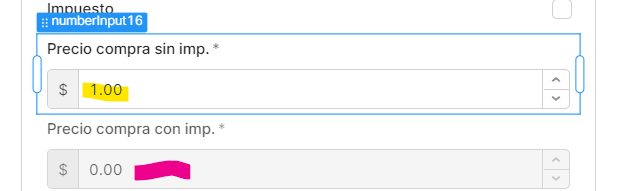# How calculate field value based on another field

hi i got two fieldsi would like when i write in the first field: 1.00 then in the second field auto calculate the result of 1.00 + 7% so total will be = 1.07 thats a tax thing in my country

what is the best approach for this?

thank you

In the field where you want to see the calculation with tax
it should be a number field
`{{numberInput1.value*.07 + numberInput1.value}}`

2 Likes

thank you ! works perfect